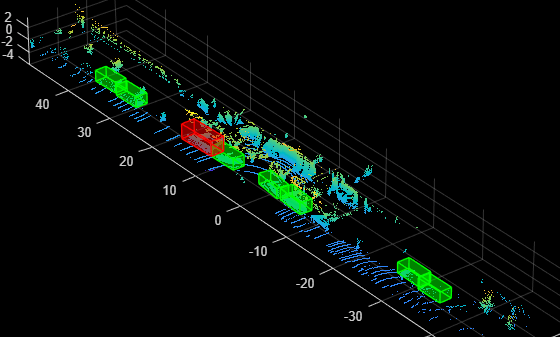# detect

Detect objects using PointPillars object detector

## Syntax

``bboxes = detect(detector,ptCloud)``
``````[bboxes,scores] = detect(detector,ptCloud)``````
``[___,labels] = detect(detector,ptCloud)``
``detectionResults = detect(detector,DS)``
``[___] = detect(___,Name=Value)``

## Description

````bboxes = detect(detector,ptCloud)` detects objects within the input point cloud, `ptCloud`. The function returns the locations of detected objects as a set of bounding boxes. ```
``````[bboxes,scores] = detect(detector,ptCloud)``` returns the class-specific confidence score for each bounding box.```
````[___,labels] = detect(detector,ptCloud)` returns the label assigned to each bounding box. The labels used for object classes are defined during training by using the `trainPointPillarsObjectDetector` function.```
````detectionResults = detect(detector,DS)` detects objects within the series of point clouds in the datastore `DS`. ```

example

````[___] = detect(___,Name=Value)` specifies options using one or more name-value arguments in addition to any combination of arguments from previous syntaxes. For example, `detect(detector,ptCloud,Threshold=0.5)` detects objects within the input point cloud with a detection threshold of `0.5`.```

## Examples

collapse all

Load a pretrained PointPillars object detector into the workspace.

```pretrainedDetector = load("pretrainedPointPillarsDetector.mat","detector"); detector = pretrainedDetector.detector;```

Read the input point cloud using the `pcread` function.

`ptCloud = pcread("PandasetLidarData.pcd");`

Run the pretrained object detector on the point cloud.

```[bboxes,scores,labels] = detect(detector,ptCloud); bboxCar=bboxes(labels'=="Car",:); bboxTruck=bboxes(labels'=="Truck",:);```

Visualize the results using the `pcshow` function. For better visualization, select a region of interest, `roi`, from the point cloud data. Display the bounding boxes for cars, trucks using the `showShape` function.

```roi = [0.0 89.12 -49.68 49.68 -5.0 5.0]; indices = findPointsInROI(ptCloud,roi); figure ax = pcshow(select(ptCloud,indices).Location); zoom(ax,1.5) showShape("cuboid",bboxCar,Color="green",Parent=ax,Opacity=0.3,LineWidth=1) hold on; showShape("cuboid",bboxTruck,Color="red",Parent=ax,Opacity=0.3,LineWidth=1)```## Input Arguments

collapse all

PointPillars object detector, specified as a `pointPillarsObjectDetector` object.

Input point cloud, specified as a `pointCloud` object. This object must contain the locations, intensities, and RGB colors necessary to render the point cloud.

Datastore, specified as a valid datastore object, which is a collection of point clouds. This datastore must be set up such that using the `read` function on the datastore object returns a cell array or table, the first column of which contains point clouds. For more information on creating datastore objects, see the `datastore` function.

### Name-Value Arguments

Specify optional pairs of arguments as `Name1=Value1,...,NameN=ValueN`, where `Name` is the argument name and `Value` is the corresponding value. Name-value arguments must appear after other arguments, but the order of the pairs does not matter.

Example: `detect(detector,ptCloud,Threshold=0.5)`

Detection threshold, specified as a scalar in the range [0, 1]. The function removes detections that have scores lower than this threshold value. To reduce false positives, increase this value.

Strongest bounding box selection for each detected object, specified as a logical `1` (`true`) or `0` (`false`).

• `true` — The function returns the strongest bounding box per object. The function uses the `selectStrongestBboxMulticlass` function, which uses nonmaximal suppression to eliminate overlapping bounding boxes based on their confidence scores.

By default, `detect` uses this code for the `selectStrongestBboxMulticlass` function:

``` selectStrongestBboxMulticlass(bbox,scores,RatioType="Union", ... OverlapThreshold=0.1);```

• `false` — The function returns all the detected bounding boxes. You can then use a custom process to eliminate overlapping bounding boxes.

Size of mini-batch, specified as a positive scalar. Use the `MiniBatchSize` argument to process a large collection of point clouds. Using this argument, the function groups point clouds into mini-batches and processes them as a batch to improve computational efficiency. Increase the mini-batch size to decrease processing time. Decrease the size to use less memory.

Performance optimization, specified as `"auto"` or `"none"`.

• `"auto"` — Automatically selects the optimizations suitable for the network and environment of the detector. These optimizations improve performance at the expense of some overhead on the first call and possible additional memory usage.

• `"none"` — Disables all acceleration.

## Output Arguments

collapse all

Locations of the objects detected within the point cloud, returned as an M-by-9 matrix. Each row in the matrix is of the form [x y z length width height roll pitch yaw], representing the dimension and location of the bounding box. M is the number of bounding boxes.

Detection confidence scores for the bounding boxes, returned as an M element column vector. M is the number of bounding boxes. The score for each detection is the product of its objectness prediction and classification scores. Each score is in the range [0, 1]. A higher score indicates higher confidence in the detection.

Labels for bounding boxes, returned as an M-by-1 categorical array. M is the number bounding boxes in the point cloud. Define the class names used to label the objects when you train the object detector.

Detection results, returned as a table with columns, `Boxes`, `Scores`, and `Labels`. Each row of the table corresponds to a point cloud from the input datastore.

Column NameValueDescription
BoxesM-by-9 matrix, where M is the number of bounding boxes.Bounding boxes for objects found in the corresponding point cloud.
Scores M element column vectorDetection scores for the bounding boxes.
LabelsM-by-1 categorical arrayLabels for the bounding boxes.

To evaluate the detection results, use the `evaluateDetectionAOS` function.

`metrics = evaluateDetectionAOS(detectionResults,testLabels);`

## Version History

Introduced in R2021b